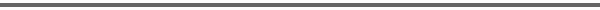Journal Home Page Complete Contentsof this Volume Previous Article Next Article Journal of Lie Theory 14 (2004), No. 1, 165--198 Copyright Heldermann Verlag 2004Classification des Structures CR Invariantes pour les Groupes de Lie Compacts Jean-Yves Charbonnel Universite Paris 7 - CNRS, Institut de Mathématiques de Jussieu, Théorie des groupes, Case 7012, 2 Place Jussieu, 75251 Paris Cedex 05, France, jyc@math.jussieu.fr Hella Ounaďes Khalgui Université de Tunis, Faculté des Sciences, Département de Mathématiques, Campus Universitaire, 1060 Tunis, Tunisia, o.khalgui@fst.rnu.tn[Abstract-pdf] Let $G_0$ be a compact Lie group of dimension $N$ whose Lie algebra is ${\frak g}_0$. The notion of $CR$ structure on a C$^{\infty }$ manifold is known a long time ago. In this note we are interested by the $CR$ stuctures on $G_0$ which are invariant by the left action of the group on the tangent bundle and which are of maximal rank. Such a structure is defined by its fibre ${\frak h}$ at the neutral element which is a subalgebra of the complexification ${\frak g}$ of ${\frak g}_0$ whose dimension is the entire part $[N/2]$ of $N/2$ and whose intersection with ${\frak g}_0$ is equal to $\{0\}$. Up to conjugation by the adjoint group of ${\frak g}_0$, these subalgebras are classified. When $N$ is even, there is only one type, type $CR0$. When $N$ is odd, there are two types, type $CR0$ and type $CRI$. These types are given in terms of Cartan subalgebras and root systems. In any case, these subalgebras are solvable. Following M. S. Baouendi, L. P. Rothschild and F. Treves ["CR structures with group action and extendability of CR functions", Inventiones Mathematicae 82 (1985) 359--396], we introduce the notion of $CR$ structures which are $G_0$-invariant and invariant by the transverse action of a $G_0$-invariant Lie subgroup. When this group is commutative, we get the notion of $G_0$-rigidity. We then prove, when $N$ is odd, that a $G_0$-invariant $CR$ structure, of maximal rank, is $G_0$-rigid if and only if the fibre of the $CR$ structure at the neutral element, is of type $CR0$. Following H. Jacobowitz ["The canonical bundle and realizable CR hypersurfaces", Pacific Journal of Mathematics 127 (1987) 91--101], we introduce the canonical fibre bundle $K$ of a $G_0$-invariant $CR$ structure, of maximal rank, when $N$ is odd. We prove that $K$ contains a closed $G_0$-invariant form if and only if the fibre of the $CR$ structure at the neutral element, is of type $CR0$ or type $CRII$. As for type $CR0$ and $CRI$, the $CRII$ type is defined up to conjugation by the adjoint group of $\g_0$ in terms of Cartan subalgebras an root systems. In fact, every subalgebra of type $CRII$ is of type $CRI$. [ Fulltext-pdf  (309  KB)] for subscribers only.